Journal of Investment and Management
Volume 5, Issue 6, December 2016, Pages: 184-188

Empirical Analysis of Fuzzy Synthetic Evaluation of Enterprises’ Financial Risks

Institute of Datong Securities Asset Management, Yongbao Ji*

Department of Asset Management, Datong Securities, Shanghai, China(Yongbao Ji)

Institute of Datong Securities Asset Management: Jian Fan, Chun Li, Yongbao Ji, Yang Bin Peng, Jiaqi Shen;

*Corresponding author

Institute of Datong Securities Asset Management, Yongbao Ji. Empirical Analysis of Fuzzy Synthetic Evaluation of Enterprises’ Financial Risks. Journal of Investment and Management. Vol. 5, No. 6, 2016, pp. 184-188. doi: 10.11648/j.jim.20160506.22

Received: November 21, 2016; Accepted: December 12, 2016; Published: December 28, 2016

Abstract: It is very important for enterprises to know how much financial risks themselves can bear, which is benefit for enterprises to avoid financial risks turning into financial crisis. How to recognize and evaluate financial risks become the most significant problem when operate enterprises. At the same time, make good use of financial risks also become an important content of the risk management object. The paper builds up financial risks index system of enterprises, which is feasible by using the fuzzy synthetic evaluating model. This method make up for tradition method pay more attention to quantitative index, and the accuracy is better than the tradition method. Moreover, this method can adjust the index system and the weight to adapt different industry, and also have the expansion.

Keywords: Enterprises Financial Risks, Analysis Hierarchy Process, Fuzzy Synthetic Evaluation

1. Introduction

Nowadays, economic globalization has become an important symbol of the development of the world economy. With the continuous improvement of China's market economy system, the expansion of the financial system, the external disturbance to China's financial market has become more and more obvious. With the economic globalization of economic and financial market, it has brought more opportunities to the enterprise for development. But the risks and opportunities coexist. It is more concerned and worried about the financial risks of enterprises. Financial development is directly related to the development of enterprises. The severe situation of financial environment put forward higher request to the enterprise financial risk management.

Enterprise financial risk refers to the possibility of economic losses due to the changes of the basic financial variables such as exchange rate, interest rate and stock price in a certain period of time when the enterprise is engaged in financial activities . The financial risk is mainly the interest rate volatility, exchange rate volatility and the risk of price fluctuations. Its basic feature is different from the business risk. The basic content of enterprise financial risk management is to determine the enterprises’ financial risk management objectives, to make the enterprises’ financial risk to achieve the goal state.

Traditional financial risk assessment model is univariate model evaluation, multivariable linear model evaluation, probability model evaluation and so on . Most of the traditional models are based on quantitative indicators, using very few qualitative indicators . Modern enterprise management pay more attention to individual components, leadership, staff comprehensive ability and other qualitative indicators and the interest rate volatility, exchange rate volatility and the risk of price fluctuations. If we ignore the use of qualitative indicators, the decision will not be comprehensive. Also, financial risk assessment indicators are numerous, these indicators are closely linked. How to find out the main index of financial risk is the key to assess the financial risk. We attempt to quantify the uncertain qualitative index based on the fuzzy mathematics theory.

Combined with the comprehensive evaluation index system of enterprise financial risk, The paper build up financial risks index system to evaluate financial risks by using the fuzzy synthetic evaluating model which makes up for tradition method pay more attention to quantitative index. Also we can adjust the index system and the weight to adapt different industry.

2. Fuzzy Synthetic Evaluate Financial Risks

The traditional models of evaluating financial risks are single variable model evaluating, multivariate linear model evaluating, probability model evaluating and so on. Most of the traditional models are on the foundation of quantitative indexes, seldom qualitative indexes are used. The modem enterprise management pays more attention to qualitative index such as the ingredient of individual, the capability of leader, the employee’s comprehensive capability, etc. If ignore the usage of qualitative indexes, the decision will be not comprehensive. Secondly, the indexes of financial risk evaluation are numerous, and there are close contacts in those indexes. How to find out the main indexes of influence financial risk, is the key that how to evaluate the financial risk.

According to above respect, this paper tries to take fuzzy mathematics theories as foundation, and make qualitative index which range is unclear in enter risk quantified by the way of fuzzy mathematics. Combine the comprehensive evaluation index system of the enterprise financial risks, and make use of the Analysis Hierarchy Process to fuzzy synthetic evaluate financial risk from the angle of paying ability, profit-gaining capacity, etc.

Chose Financial Risk Evaluating Index

This paper holds the view that financial risks evaluating index should include: profit-gaining capacity, paying capacity, property management capacity, growth capacity, cash capacity, and basic risk conditions .

Basic capacity index: paying capacity. On account of the financial risks main expression is the possibility of enterprise call not payback matured liabilities. The basic aim of financial risks control is guarantee the capacities of payback matured liabilities, and guarantee the capacity of reproduction. Paying capacity is the direct guarantee of financial risks.

Auxiliary capacity index: profit-gaining capacity and property management capacity. Profit-gaining is the main guarantee of enterprise which bears risks. When the enterprise gets loss in business, the loss should be remedied by profit. If the profit can not remedy the loss, the capital will be loosed. Moreover, a better capital structure is good to financial risks control. All of this needs the high level of capital management. So, we should use auxiliary capacity index in financial risks index.

Assurance capacity index: cash capacity and growth capacity. In the operation of the enterprise, cash play an important part, looks like the blood of the business . Moreover, if the enterprise wants grow bigger, enterprises can't only consider its short-term development, still need to pay attention to the long-term development.

Qualitative capacity index: basic risk condition, such as the professional level of the accountant, the liquidity of the accountant, financial risks consciousness of leader. With the expanse of the enterprise scale, these ingredients not only reflect the basic financial risk of enterprise, but also affect financial activity and financial decision.

In order to effectively evaluating the financial risks, simultaneously gives dual attention to representation, comparison, collecting and concision, we uses six types of indices as following. The first type, index of profit-gaining capacity: main business profit margin, asset profit margin, main business gross profit margin; the second type, index of

Paying Capacity: balance sheet ratio, quick ratio, interest earned ratio; the third type, index of growth capacity: capital growth ratio, sales growth ratio, retained earnings to capital ratio ; the fourth type, index of growth capacity: cash to current debt ratio, cash to main business profit ratio, cash to capital ratio; the fifth type, index of property management capacity: turnover of capital ratio, Turnover of receivables ratios; the sixth type, index of basic risk condition: liquidity of accountants, consciousness of financial risk .

Fuzzy Synthetic Evaluating Model in Evaluating of Financial Risks

The analytic model based on BP neural network assumes the data used in modeling to be a sequence.

For the observation value of sequence p, formula (1) and (2) are the model output value of sequence k and the optimized index (sum of error square), and formula (3) is the prompting function.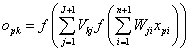k=1,…m      (1)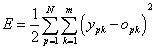(2)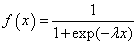(3)

The function of multi-input and multi-output is the feed-forward process. Step 1 is to calculate a value through the linear combination of the input value and the weight value wji. Step 2 is to calculate a mid-value through putting the value into (3). Step 3 is to calculate another value through another linear combination of the mid-value and the weight value vkj. Step 4 is to calculate the output of the model through putting this value into (3). All steps are shown in formula (1). And formula (4) is the sum of error square of sequence p.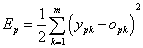(4)

Error back-forward is that the output error scale between the expected and the model determines the weight increasing scale between the hidden layer and the output layer. The weight increasing scale between the input layer and the hidden layer is determined in the same way . By analogy, this method fits if it is multilayer.

The training process is:

STEP1: Chosen initial weight value (stochastic or man-made);

STEP2: Calculating the output of the model (Opk) and the result of the complex functions (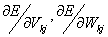);

STEP3: Modifying weight values by formula (5) and (6) as=1;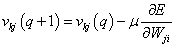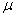>0(5)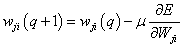>0(6)

STEP4: Doing circularly until constringency or the iterative-time (q) reaching a certain value.

According to the model above, {x1,x2…xn} is a set of observation values or anticipation values for input, while {y1,y2…ym} is a set of observation values or forecast values for output. In this research, the indexes of the input and output data are the relevant value of those indexes shown in the next section.

Financial risks evaluating index system includes so many complications, such as uncertain factors and quantitative index . The sureness of the scheme is fuzzy. The paper adopts fuzzy optimum seeking theory in select the mode of financial risk evaluating, the processes is as the follow shows.

Confirming the scheme aggregation

Supposing that there are n schemes constituting a scheme aggregation:

D = {d1, d2,..., dn,}                       (7)

Optimum seeking must be completed in the D aggregation, that is, n schemes in D aggregation have an excellent and inferior comparison, and others out of D aggregation are irrelevant. It is relativity for optimum seeking theory.

Making sure the evaluation indexes of scheme

Supposing that each scheme has m evaluation indexes, so m evaluation indexes of n schemes can be expressed by eigenvalue matrix of indexes:(8)

And i =l, 2…m; j=l, 2...n

As to the adverse index, turn it to positive index by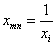As to the negative index, select a suitable value a, converse it by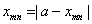.

The standardization of evaluation indexes

Because there are dimension differences between each evaluation index, in order to eliminate the influence, we must have standardizations on m indexes: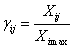(9)

And--the eigenvalue of the i index of the j scheme,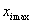--the maximum of the i index.

Then we can turn the eigenvalue matrix of evaluation indexes into relative belonging matrix :(10)

Making sure the evaluation indexes of scheme

Because m indexes play different roles on optimum seeking, we should entitle them different weight. Supposing that the weight vector of m evaluation indexes is as the follow:

W= (w1, w2,..., wm)                   (11)

And Wi is the weight of i evaluation index. There are many measures in confirming weight, such as expert offering score, analytic hierarchy process, etc. The paper adopts analytic hierarchy process.

Confirming the optimal and the worst scheme

Supposing that the optimal scheme is G =(gl,g2, …,gm), and the worst one is B = (b1,b2,...,bm), according to the relativity of fuzzy optimum seeking theory, gi and bi is as the follow: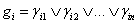(12)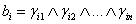(13)

In formula (12) and formula (13), gi is i index of the optimal scheme), bi is i index of the worst scheme.

For the sake of the calculation convenience, the optimal scheme is considered as G = (1, 1, …, 1), and the worst one is B = (0,0,…,0).

Compute the superior belonging degrees of the schemes

According to the follow formula, we can get the superior belonging degree Ui of the j scheme:(14)

And p is the distance parameter, and generally is 1 or 2. Then we can get the superior belonging degree of each scheme:

U= (u1, u2,…, un)                        (15)

According to the maximum superior belonging principle, we can confirm the optimal scheme and make a sequence about superior and inferior schemes.

3. Demonstrations of Financial Risks Evaluating Model

In order to demonstrate the financial risks evaluating model, we should be follow the steps are as follow.

3.1. Confirming the Scheme Aggregation of Financial Risk

This paper selects the enterprise of same industry, which is financial enterprise. Through widely analyze, we can select three which constitute a scheme aggregation U = (U1, U2, U3).

The selecting of the mode of financial risk is a system project, and we should consider all factors, such as obtain the date, and calculate the date and so on. So we choose profit-gaining capacity index, paying capacity index, property management capacity index, growth capacity index, and cash capacity index, to confirm the weight of each index .

Table 1. The evaluation index and weight of schemes.

 First Index Wei-ght Second Index Wei-ght Scheme 1 Scheme 2 Scheme 3 A 0.2 A1 0.5 0.0510262 0.1133501 0.217328 A2 0.5 0.05 0.0363183 0.1760457 B 0.3 B1 0.3 0.4927589 0.7175649 0.735447 B2 0.3 0.48 0.2874756 0.3849974 B3 0.4 6.2424827 2.0499011 7.1727907 C 0.15 C1 0.4 0.9885716 0.6306106 0.9311165 C2 0.6 9.1638145 8.7711432 11.511585 D 0.05 D1 0.4 -0.063092 0.0049698 -0.19343 D2 0.6 -0.282262 0.3177033 -0.027938 E 0.3 E1 0.5 0.9215518 0.4501319 0.6245126 E2 0.5 0.2295556 0.2302592 0.2444833

Table 1 shows the evaluation index and weigh of schemes. Those letters mean some indexes. A: profit-gaining capacity Index, A1: main business profit margin; A2: asset profit margin. B: paying capacity index, Bl: balance sheet ratio; B2: quick ratio; B3: interest earned ratio. C: property capacity index, C1: turnover of capital ratio; C2: of receivables ratio. D: growth capacity index, D1: growth capital ratio; D2: growth of sales ratio. E: cash capacity index, El: Cash to current debt ratio; E2: main business profit ratio.

3.2. The Standardization of Evaluation Indexes

According to formula (8) and (9), we can turn the evaluation indexes in Table 1 into relative belonging degrees. The result is as Table 2.

3.3. Confirming the Weight of Evaluation Indexes

The paper adopts analytic hierarchy process, and the concrete process no longer goes into detail. The result is as Table 2.

Computing the superior belonging degrees of the schemes

Firstly, we can carry on the optimization of the first floor time, and constitute relative superiors belonging degrees of tow indexes of profit-gaining capacity index into a matrix of superior belonging degrees of evaluation indexes in Table 2.

Table 2. The relative belongging degree and weight of evaluation indexes of schems.

 First Index Wei-ght Second Index Wei-ght Scheme 1 Scheme 2 Scheme 3 A 0.2 A1 0.5 1 0.4501649 0.234789 A2 0.5 0.7580416 1 0.2063002 B 0.3 B1 0.3 0.6700128 0.9756854 1 B2 0.3 0.5962692 1 0.746695 B3 0.4 0.3283791 1 0.2857885 C 0.15 C1 0.4 0.6379008 1 0.6772628 C2 0.6 0.9571498 1 0.7619406 D 0.05 D1 0.4 0.8608853 0.8025818 1 D2 0.6 1 0.5446883 0.7383663 E 0.3 E1 0.5 0.4884499 1 0.720773 E2 0.5 1 0.9969442 0.9389419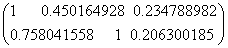We can know from Table 2 that the weights of five indexes of profit-gaining capacity index are:

W= (0.5 0.5)

We can know that the optimal scheme and the worst one are G= (1, 1, …, 1) and B= (0, 0, …, 0), and the value of P is 2, the result is as follows:

U1 = (0.949182191 0.861414353 0.070808352)

Then we can calculate the superior belonging degrees of schemes in paying capacity indexes:

U2 = (0.948051194 1 0.883966892)

U3 = (0.993571483 0.721853998 0.935305345)

U4 = (0.993571483 0.721853998 0.935305345)

U5 = (0.880888877 0.999993256 0.96205161)

Then constituting the first evaluation result into a relative superior belonging degree matrix of the second evaluation indexWe can learn from Table 2 that the weights of paying capacity and property management capacity are:

W= (0.2, 0.3, 0.15, 0.05, 0.3)

According to the formula (8), we can get the result:

U = (0.853426724, 0.995897658, 0.748958831)

As result, the superior belonging degree of the first, second and third scheme are 0.853, 0.996, 0.749. According to the principle of the maximum belonging degree, we can learn that the second scheme is the optimum.

3.4. Analyze the Result of Evaluation

We can draw the conclusion from the interrelation of each index, the financial risks of U2 enterprise came from the debt risk, the balance sheet ratio of the enterprise is nearly 72%, but the high balance sheet ratio is not guaranteed by high main business profit margin. The enterprise can not make good use of financial leverage, so the financial risk heightened. At the same time, the property management capacity and the cash capacity of U2 enterprise is also relatively lower, so the financial risk exist.

4. Conclusions

The paper build up financial risks index system, it is feasible to evaluate financial risks by using the fuzzy synthetic evaluating model. This method make up for tradition method pay more attention to quantitative index, and the accuracy is better than the tradition method. Moreover, this method can adjust the index system and the weight to adapt different industry, and also have the expansion. The model also exist some deficiency in application, for example, not take good consideration in each index. And the calculation is complexity. So there should be some improvements in our future research.

References

1. F. Robert, M. Daniel, and C. Daniel, "On the linkage between financial risk tolerance and risk aversion," Journal of Financial Research, Vol. 31 Issue 1, pp.1-23, Spring 2013.
2. Li Y, Chow S, Choi M, et al. Supplier integration, green sustainability programs, and financial performance of fashion enterprises under global financial crisis[J]. Journal of Cleaner Production, 2016, 135: 57-70.
3. B. Porteous and P. Tapadar, "Economic Capital and Financial Risk Management for Financial Services Firms and Conglomerates", Journal of the Royal Statistical Society, Vol. 170 Issue 2, pp.510-510, Apr 2014.
4. Gill, I. S., Kharas, H. J., Bhattasali, D., "An East Asian Renaissance: Ideas for Economic Growth", World Bank Publications, 2007.
5. Liu R, Wang D. The ruin probabilities of a discrete-time risk model with dependent insurance and financial risks[J]. Journal of Mathematical Analysis and Applications, 2016, 444(1): 80-94.
6. Hsieh, C. T., Klenow, P. J., "Misallocation and Manufacturing TFP in China and India", The Quarterly Journal of Economics, 2009, 124(4): 1403-1448.
7. Lichtenberg, F., de la Potterie, B. P., "International R&D spillovers: A Re-examination", National Bureau of Economic Research, 1996.
8. Xouridas S. Agricultural Financial Risks Resulting from Extreme Events[J]. Journal of Agricultural Economics, 2015, 66(1): 192-220.
9. Midrigan, V., Xu, D. Y., "Finance and Misallocation: Evidence from Plant-level Data", American Economic Review, 2014(104): 422-458.
10. CHENG W, Hong H, Scheinkman J A. Yesterday's heroes: compensation and risk at financial firms[J]. The Journal of Finance, 2015, 70(2): 839-879.

 Contents 1. 2. 3. 3.1. 3.2. 3.3. 3.4. 4.
Article ToolsAbstractPDF(192K)